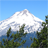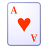cancel
Showing results for
Did you mean:

679 ViewsContributor II

I am working off AN4759 in porting the bootloader for the 56F84789 DSC to a 56F84550 DSC. I was able to compile the application but I am unable to download to the DSC. I am able to load my own program to the DSC and operation is fine. Therefore I know it cannot be the DSC that is the problem. The error that i get is "Failed to resume target process., Unknown Error (Empty text)".

I have a feeling it could be related to the linker file. As per the instructions I have disabled auto generation of the linker command file and have edited it by hand. Unfortunately the datasheet for the 56F84550 DSC on freescale.com does not have the correct information for the memory map - it is simply a copy and paste of the 56F84789 DSC. For the 56F84789 DSC there are 2K words for xRAM_bss (bss), 0.5K words for xRAM_data (global variables) and 5.5K words for xRAM (heaps and stacks). For the 56F84550 DSC however there is only 4K total compared to 8K for the 56F84789 DSC. How do I split it up correctly? The same goes for the pRAM_code - only 4K compared to 8K for the 56F84789. How to split it up correctly? The linker file is attached below. If the linker file looks ok let me know.

## ###################################################################

##

## ###################################################################

MEMORY {

.pIntvectorBoot(RWX): ORIGIN = 0x00000000, LENGTH = 0x000000F0 # Reset and cop vectors

.pFlashConfig  (RWX): ORIGIN = 0x00000200, LENGTH = 0x00000008 # Reserved for Flash IFR value

#84550

.ppFlash       (RWX): ORIGIN = 0x00000208, LENGTH = 0x0000B1F5    # 0x208 + 0xB1F5 = 0xB3FD (start of delay time)

.DelayT         (RWX): ORIGIN = 0x0000B3FD, LENGTH = 0x00000003 # Bootloading delay time & user code start position

.pFlash         (RWX): ORIGIN = 0x0000B400, LENGTH = 0x00000C00 # Primary flash for bootloader, 3Kwords

.xRAM_bss      (RW) : ORIGIN = 0x00000000, LENGTH = 0x00000500 #  bss (unitialized data)

.xRAM_data     (RWX): ORIGIN = 0x00000500, LENGTH = 0x00000100 # global variables

.xRAM           (RW) : ORIGIN = 0x00000600, LENGTH = 0x00000A00 #   heaps and stacks

.pRAM_code       (RWX): ORIGIN = 0x00061000, LENGTH = 0x00001000 # 1Kwords for code

.xRAM_code     (RW) : ORIGIN = 0x00001000, LENGTH = 0x00001000 # mirror of .pRAM_code

}

KEEP_SECTION { interrupt_vectors.text,interrupt_vectors.text }

FORCE_ACTIVE { Ffisr_sci_rx_full,Frx_data }

SECTIONS {

.interrupt_vectors :

{

# interrupt vectors

* (interrupt_vectors.text)

} > .pIntvectorBoot

.delay_time :

{

#WRITEW(0x0001F400);    # remember to change this value if different chip is used

WRITEW(0x0000B400);  # 84550

WRITEH(10);            # delay time, in seconds

} > .DelayT

.FlashConfig :

{

WRITEH(0xFFFF);    # 0, back door key

WRITEH(0xFFFF); # 1, back door key

WRITEH(0xFFFF); # 2, back door key

WRITEH(0xFFFF); # 3, back door key

WRITEH(0xFFFF); # 4, p-flash protection bits

WRITEH(0xFFFF); # 5, p-flash protection bits

#        WRITEH(0xFFFF);    # 6, opt register and security byte

WRITEH(0xFFBE);    # 6, opt register and security byte         0x040D (FOPT) & 0x040C (FSEC)

WRITEH(0xFFFF); # 7, eeprom and d-flash protection bits        0x040F (FDPROT) & 0x040E (FEPROT). Datasheet description is not in order

} > .pFlashConfig

.ApplicationCode :

{

_pFlash_code_start  = .;

# Note: The function _EntryPoint should be placed at the beginning

#       of the code

OBJECT (F_EntryPoint, Cpu_c.obj)

# Remaining .text sections

* (rtlib.text)

* (startup.text)

* (fp_engine.text)

* (user.text)

* (.text)

# save address for the data starting in pROM

Fpflash_mirror = .;

Fpflash_index  = .;

} > .pFlash

.data_in_x_ram_bss :

{

# .bss sections

_START_BSS = .;

OBJECT (Frx_data, Buffer_c.obj)

* (rtlib.bss.lo)

* (rtlib.bss)

* (.bss.char)         # used if "Emit Separate Char Data Section" enabled

* (.bss)

_END_BSS   = .;

F_Xbss_length = _END_BSS - _START_BSS;

} > .xRAM_bss

.prog_in_p_flash_ROM : AT(Fpflash_mirror)

{

Fpram_start = .;

_pram_start = .;

* (interrupt_vectors.text)

* (pram_code.text)

# save data end and calculate data block size

Fpram_end = .;

Fpram_size = Fpram_end - Fpram_start;

_pram_size = Fpram_size;

Fpflash_mirror2 = Fpflash_mirror + Fpram_size;

Fpflash_index = Fpflash_mirror + Fpram_size;

} > .pRAM_code

.data_in_p_flash_ROM : AT(Fpflash_mirror2)

{

. = ALIGN(2);

Fxram_start = .;

* (SciDataSection.data)

* (.const.data.char)    # used if "Emit Separate Char Data Section" enabled

* (.const.data)

* (fp_state.data)

* (rtlib.data)

* (.data.char)          # used if "Emit Separate Char Data Section" enabled

* (.data)

# save data end and calculate data block size

Fxram_end = .;

Fxram_size = Fxram_end - Fxram_start;

Fpflash_index = Fpflash_mirror2 + Fxram_size;

} > .xRAM_data

.data_in_x_ram :

{

/* Setup the HEAP address */

. = ALIGN(4);

_HEAP_SIZE = 0x0000;

. = _HEAP_END;

/* SETUP the STACK address */

_min_stack_size = 0x0200;

. = _stack_end;

// store page buffer

* (flash_page.page)

__DATA_END=.;

} > .xRAM

# Export HEAP and STACK to runtime libraries

F_heap_end     = _HEAP_END;

# runtime code __init_sections uses these globals:

F_Ldata_size     = 0;

F_xROM_to_xRAM   = 0x0000;

F_pROM_to_xRAM   = 0x0000;

F_start_bss      = _START_BSS;

F_end_bss        = _END_BSS;

F_pflash_code_start = Fpflash_mirror;

F_dram_code_start = _pram_start - 0x60000;

F_dram_code_size  = _pram_size;

F_pflash_data_start = Fpflash_mirror2;

F_dram_data_start = Fxram_start;

F_dram_data_size  =  Fxram_size;

F_pflash_code_start_h = (F_pflash_code_start/65536)&0xffff;

F_pflash_code_start_l = F_pflash_code_start &0xffff;

F_dram_code_start_h = (F_dram_code_start/65536) &0xffff;

F_dram_code_start_l = F_dram_code_start &0xffff;

F_pflash_data_start_h = (F_pflash_data_start/65536)&0xffff;

F_pflash_data_start_l = F_pflash_data_start &0xffff;

F_dram_data_start_h = (F_dram_data_start/65536) &0xffff;

F_dram_data_start_l = F_dram_data_start &0xffff;

}

Labels (1)
• General